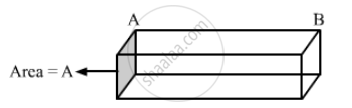Advertisement Remove all ads

# A Metal Rod of Cross Sectional Area 1.0 Cm2 is Being Heated at One End. at One Time, the Temperatures Gradient is 5.0°C Cm−1 at Cross Section a and is 2.5°C Cm−1 - Physics

Sum

A metal rod of cross sectional area 1.0 cm2 is being heated at one end. At one time, the temperatures gradient is 5.0°C cm−1 at cross section A and is 2.5°C cm−1 at cross section B. Calculate the rate at which the temperature is increasing in the part AB of the rod. The heat capacity of the part AB = 0.40 J°C−1, thermal conductivity of the material of the rod = 200 W m−1°C−1. Neglect any loss of heat to the atmosphere

Advertisement Remove all ads

#### Solution

Let the temperaturLet the temperatures at the ends A and B be TA and TB, respectively.

Rate of flow of heat at end A of the rod is given by

(dQ_A)/(dr)= KA. d/dt (T_A)

Rate of flow of heat at end B of the rod is given by

(dQ_B)/dt ( T_B)

Heat absorbed by the rod = ms∆T
Here, s is the specific heat of the rod and ∆T is the temperature difference between ends A and B.

Rate of heat absorption by the rod is given by

(dQ)/dt =ms (dT)/(dr)

∴  ms (dT)/(dr)=  (KA. dT_A
)/(dl)

⇒ (0.4). (dT)/ dt= 200 xx 1xx 10^-4xx (5-2.5)

dt/dr=12.5^circ C/sec

Concept: Thermal Expansion of Solids
Is there an error in this question or solution?
Advertisement Remove all ads

#### APPEARS IN

HC Verma Class 11, Class 12 Concepts of Physics Vol. 2
Chapter 6 Heat Transfer
Q 18 | Page 99
Advertisement Remove all ads
Advertisement Remove all ads
Share
Notifications

View all notifications

Forgot password?